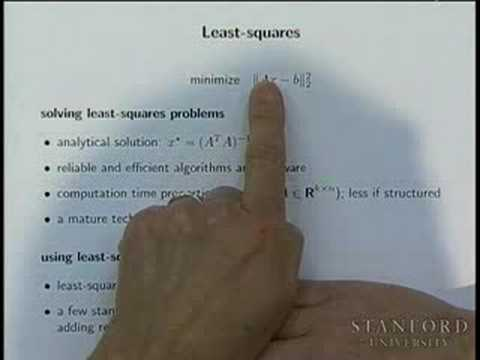Stanford Engineering

# Convex Optimization I

This course concentrates on recognizing and solving convex optimization problems that arise in engineering. Convex sets, functions, and optimization problems. Basics of convex analysis. Least-squares, linear and quadratic programs, semidefinite programming, minimax, extremal volume, and other problems. Optimality conditions, duality theory, theorems of alternative, and applications. Interiorpoint methods. Applications to signal processing, control, digital and analog circuit design, computational geometry, statistics, and mechanical engineering. (from see.stanford.edu )

Instructor

Electronics
2008

#### Lecture 01 - Introduction